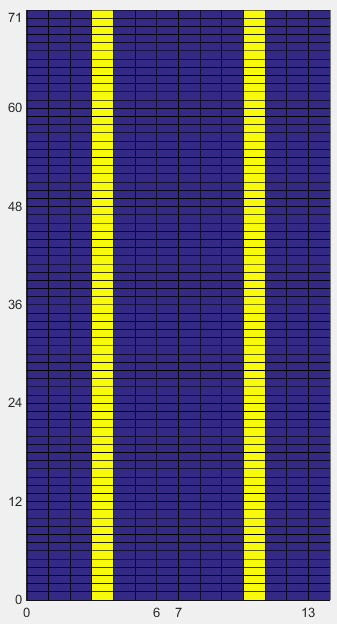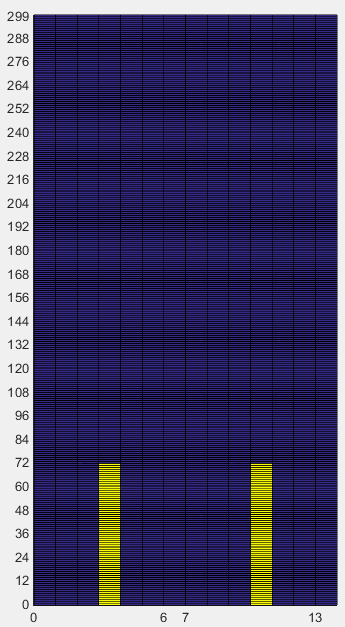MatLab Toolbox - LTE                                      Home : www.sharetechnote.com

PUSCH DMRS

Description/explanation will come later !

< Generation of PUSCH DMRS Symbol >

ue1.NCellID = 0;

ue1.CyclicPrefixUL = 'Normal';

ue1.NSubframe = 0;

ue1.Hopping = 'Off';

ue1.NULRB = 25;

ue1.Shortened = 0;

ue1.NTxAnts = 1;

ue1.SeqGroup = 0;

ue1.CyclicShift = 0;

ue1.RNTI = 1;

pusch.PRBSet = (0:5).';

pusch.Modulation = 'QPSK';

pusch.RV = 0;

pusch.DynCyclicShift = 0;

pusch.NLayers = 1;

pusch.OrthCover = 'Off';

pusch_dmrs_sym = ltePUSCHDRS(ue1,pusch);

pusch_dmrs_sym_arrayIndex = 0:length(pusch_dmrs_sym)-1;

pusch_dmrs_sym_ind = ltePUSCHDRSIndices(ue1,pusch);

subplot(1,3,1);

plot(real(pusch_dmrs_sym),imag(pusch_dmrs_sym),'ro','MarkerFaceColor',[1 0 0]);

title('Constellation');

subplot(1,3,[2 3]);

plot(pusch_dmrs_sym_arrayIndex,real(pusch_dmrs_sym),'ro-',pusch_dmrs_sym_arrayIndex,imag(pusch_dmrs_sym),'bo-');

xlim([0 max(pusch_dmrs_sym_arrayIndex)]);

title('PUSCH DMRS index vs PUSCH DMRS value. Red -> real, Blue -> Imaginary');

 ue1.NCellID = 0; ue1.NSubframe = 0; ue1.NULRB = 6; ue1.Shortened = 0; ue1.NTxAnts = 1;   pusch.PRBSet = (0:5).'; pusch.Modulation = 'QPSK'; pusch.RV = 0; pusch.DynCyclicShift = 0;< Symbol to RE Mapping >

ue1.NCellID = 0;

ue1.CyclicPrefixUL = 'Normal';

ue1.NSubframe = 0;

ue1.Hopping = 'Off';

ue1.NULRB = 25;

ue1.Shortened = 0;

ue1.NTxAnts = 1;

ue1.SeqGroup = 0;

ue1.CyclicShift = 0;

ue1.RNTI = 1;

pusch.PRBSet = (0:5).';

pusch.Modulation = 'QPSK';

pusch.RV = 0;

pusch.DynCyclicShift = 0;

pusch.NLayers = 1;

pusch.OrthCover = 'Off';

pusch_dmrs_sym = ltePUSCHDRS(ue1,pusch);

pusch_dmrs_sym_arrayIndex = 0:length(pusch_dmrs_sym)-1;

pusch_dmrs_sym_ind = ltePUSCHDRSIndices(ue1,pusch);

resourceGridUL = lteULResourceGrid(ue1);

pusch_dmrs_scale = 0.5;

resourceGridUL(pusch_dmrs_sym_ind) = pusch_dmrs_scale * pusch_dmrs_sym;

resourceGridUL = [ resourceGridUL resourceGridUL(:,13)];

resourceGridUL = [ resourceGridUL; resourceGridUL((ue1.NULRB*12-1),:) ];

xStep = 0:14;

yStep = 0:(ue1.NULRB*12);

surface(xStep,yStep,abs(resourceGridUL));

axis([0 14 0 (ue1.NULRB*12) 0 2]);

view([0,90]);

set(gca,'xtick',[0 6 7 13]);

set(gca,'ytick',[[0:12:ue1.NULRB*12-1] [ue1.NULRB*12-1]]);

 ue1.NCellID = 0; ue1.NSubframe = 0; ue1.NULRB = 6; ue1.Shortened = 0; ue1.NTxAnts = 1;   pusch.PRBSet = (0:5).'; pusch.Modulation = 'QPSK'; pusch.RV = 0; pusch.DynCyclicShift = 0; ue1.NCellID = 0; ue1.NSubframe = 0; ue1.NULRB = 25; ue1.Shortened = 0; ue1.NTxAnts = 1;   pusch.PRBSet = (0:5).'; pusch.Modulation = 'QPSK'; pusch.RV = 0; pusch.DynCyclicShift = 0;Disclaimer ! :

This page is only to show you the overall logics and visualization for various LTE physical layer channels. I haven't investigated much about verifying about the accuracy.

If you think the code is not so efficient, it is 100% my fault. I haven't made any effort for effiecient code. I just tried to create code as simple as possible for the readers. As you know, easy-to-read code is not always efficient for a specific chipset.

If you find any mistake in terms of accuracy, it is also very highly likely be my fault. Not the problem of Matlab tool box itself.

Any comment and corrections if you find any mistake will be welcome and appreciated.